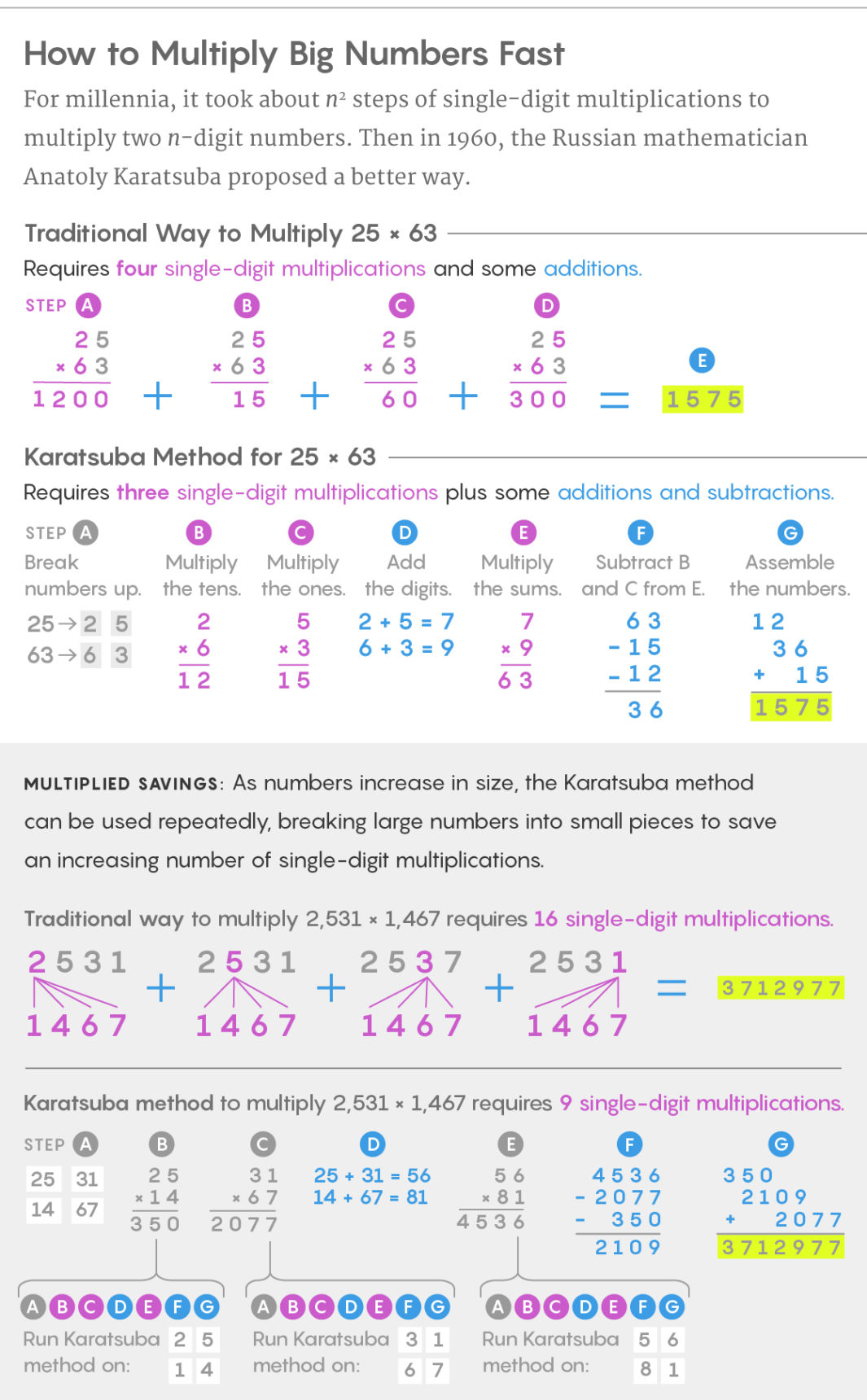# aliquot

## < a quantity that can be divided into another a whole number of time />

Some not so random links found on Twitter or elsewhere that I haven’t had time to put on the Micro section.

• Migrating from Gmail to FastMail (part 2): My Gmail became my very own garbage account, where almost every incoming mail is guaranteed to end up in the trash can, and I am aware many others switched over Fastmail or Proton, or are running their own mail server. I was surprised to learn that such move did occur as soon as 2013, as exemplified in this interesting blog post.

• Unfortunately, I lost the reference on Twitter, but I happened to see this link a few days ago: Normal samples ith linear constraints and given variances (PDF, 4 pp.). The author describes an algorithm to generate normal variates $x_1, x_2,\dots, x_n$ satisfying $k$ linear and independant relations $\sum_{j=1}^n a_{pj}x_j=0$ ($p=n-k+1,\dots,n$) with a given variance structure $\text{var}(x_i)=v_i$, $i=1,2,\dots,n$.

• Perhaps you have seen the controversy about the precedence of mathematical operators to calculate, for example, 6/2(2+1). In this case, do you think the result should be 9 or 1? Julia returns 1 while I would have said 9. Here is why: Clarify precedence of numeric literal coefs over parenthesis.

• Tries are interesting sorted-sequence data structure in that they are designed specifically for string keys. Indeed, tries are trees whose edges are labeled by characters or strings, and the characters along a root-leaf path represent a key. Using appropriate data structures for the inner nodes, a trie can be searched in time $\mathcal{O}(s)$ for a string of size $s$.1 There is a tight connection to radix sort. The hypertrie project provides a distributed single writer key/value store in javascript, that depends on hypercore. The latter is one of the core pilar of the dat project (see Tom MacWright’s review of the decentralized web), which I heard again recently because a guy processed several Gig of Wikipedia offline using a local dat dataset over personal wifi. Sidenote: If you are interested in trees and Racket, be sure to check Matt Might’s post on red-black trees.

• The Evolution of a ggplot: Nice wrap-up of how to customize a ggplot graphic. I personally liked the way the authors started with a basic boxplot and improve it in several directions, going so far as to include a tile map legend.

• Still some papers to read before I go back to work: 25 Years of Molecular Biology Databases: A Study of Proliferation, Impact, and Maintenance, Machine Learning and Statistical Methods for Clustering Single Cell RNA-sequencing Data (with code on Github).

• You know how happy I am with Emacs. Still I cannot resist looking at what others are actually using as their main text editors. Just recently I heard about Glamorous Toolkit, which looks like lot of fun to navigate a codebase, and even provides live and debuggable notebooks.

• In my last post on Newton’s method, I mentioned a simple algorithm to find the approximate square root of a number: Let’s say you are interested in finding the square root $x=\sqrt{a}$, $a>0$. One way to do this is to start with a reasonable first guess, $x_1>0$, and iterate with the following approximation: $x_{n+1}=\frac{1}{2}\left(x_n+\frac{a}{x_n}\right)$. This approach was first proposed by Babylonians, who also happened to invent multiplications. A nice alternative to standard multiplication is the so-called Karatsuba method, which offers a nice compromise between additions and multiplications so that overall there are less than $n^2$ steps to perform. Karatsuba approach is discussed in Don Knuth’s TAOCP, as well as in Modern Computer Algebra, by Joachim von zur Gathen & Jürgen Gerhard. Later, Schönhage and Strassen provided an even better algorithm, partly based on FFT. Finally, Harvey and van der Hoeven proposed an algorithm that allows to perform multiplication of very large numbers in $n\log(n)$ steps.• TIL the Linux Journal has reached the end of its existence. Sad news for the FOSS community.

1. Mehlhorn, K. and Sanders, P., Algorithms and Data Structures. Springer, 2010. [return]